Courses

# Fourier Analysis Of Discrete Time Signals

## 10 Questions MCQ Test Mock Test Series for Electrical Engineering (EE) GATE 2020 | Fourier Analysis Of Discrete Time Signals

Description
This mock test of Fourier Analysis Of Discrete Time Signals for GATE helps you for every GATE entrance exam. This contains 10 Multiple Choice Questions for GATE Fourier Analysis Of Discrete Time Signals (mcq) to study with solutions a complete question bank. The solved questions answers in this Fourier Analysis Of Discrete Time Signals quiz give you a good mix of easy questions and tough questions. GATE students definitely take this Fourier Analysis Of Discrete Time Signals exercise for a better result in the exam. You can find other Fourier Analysis Of Discrete Time Signals extra questions, long questions & short questions for GATE on EduRev as well by searching above.
QUESTION: 1

### The discrete timefourier transform for the given signal x[n] - u[n] is

Solution: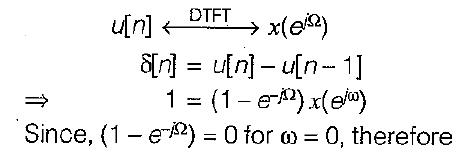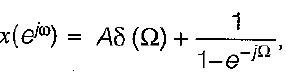where A is constant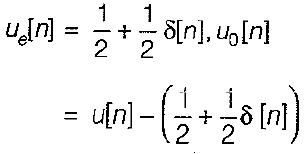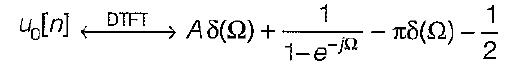The Fourier transform of odd real sequences must be purely imagenary, thus A = π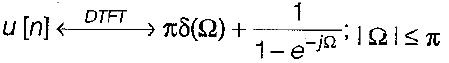QUESTION: 2

### The discrete time Fourier coefficients x[k] of the signal x [n ] =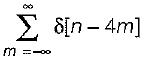Solution: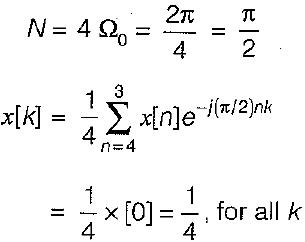QUESTION: 3

### The zero state respone y(k) for input f(k) = (0.8)k u(k) is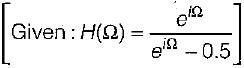Solution: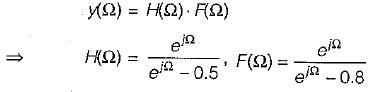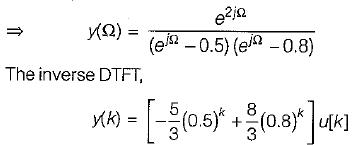QUESTION: 4

Consider discrete time sequence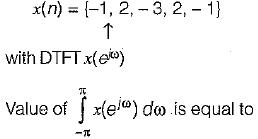Solution: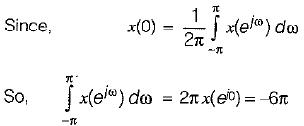QUESTION: 5

Consider a signal x(n) with following factors:
1. x(n) is real and even signal
2. The period of x(n) is N = 10
3. x(11) = 5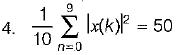The signal x(n) is

Solution: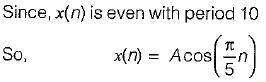QUESTION: 6

Consider a discrete time signal x(n) = {-1, 2, -3, 2, -1} value of ∠x(e) is equal to

Solution:
QUESTION: 7

A low pass filter with impulse response h1(n) has spectrum H1 (e) shown below.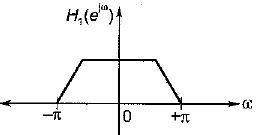Here only one period has been shown by reversing every second sign of h1(n) a new filter having impulse response  h2(t) is created. The spectrum of H2(e) is given by

Solution:
QUESTION: 8

A red signal x[n] with Fourier transform x(e) has following property:
1. x[n] = 0 for, n > 0
2. x [ 0] > 0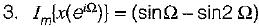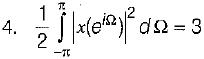The signal x[n] is

Solution:
QUESTION: 9

A causal and stable LTI system has the property that,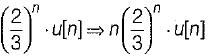The frequency response H(e) for this system is

Solution:
QUESTION: 10

A 5-point sequence x[n] is given as 4 [- 3] = 1, x[ - 2] = 1, x[ -1 ] = 0 . x = 5 , x - 1
Let x(e) denote the discrete time fourier transform of x[n].
The value of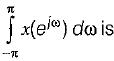Solution: